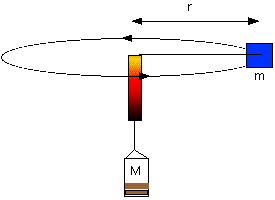Physics Honors/AP 1 and 2

#### Course Materials are always under revision! Weblecture content may change anytime prior to two weeks before scheduled chat session for content.Physics Lab

# Physics Lab: Centripetal Force

## Goal: to measure centripetal force

#### Materials

Remember that the setup below is a suggestion..

• a short (3-4") plastic (or other low-friction) tube less than 1" in diameter, which you can hold in one hand. It should be narrow and a cord sliding through it should move freely.
• a fixed mass m
• a cord or nylon string 2-4 feet in length, which can fit through the tube
• light-weight clip or marker (scotch tape?)
• standard masses (such as nickels, at 5 gr each)
• some low mass method (net bag?) of attaching standard masses into a collective mass M
• stop watch and handy fellow observer

#### Procedure

Pass the cord through the tube.

1. Attach the standard mass to one end. Record the mass of this standard.
2. Attach your bag, bucket, or other mass-collection device to the other end.
3. Place mass M greater than the standard mass m in the collection bag.
4. Mark a point on the cord between the tube and the mass-collection bag. The length of the cord from the standard mass to the marker, minus the length of the tube, will be the radius of your standard-mass orbit when the marker is just below the tube.
5. Hang onto the tube, and practice swinging your assembly so that the standard mass revolves with a reasonably steady speed. The standard mass should rise until the position of the tape marker is just below the tube.1. Add mass to the collection bag and speed up the rotation rate. Strive for constant speed.
2. Now for the data collection. Repeat the following at least 5 times, using a different mass each time in the collection bag.
1. Place a mass greater than the standard mass in the collection bag. You may wish to choose some proportional increments, but this is not necessary.
2. Start your standard mass rotating at a speed which allows the marker to rise slightly, then keep the marker at the same position. Since the tension on the cord is constant (assume no stretching), the centripetal force now equals the gravitational force.
3. Have your fellow observer time 10 rotations while you keep the marker just below the tube.
4. Repeat your observation with the same mass 3 times before changing masses.
3. You should have a total of 15 times, 3 for each of 5 masses.

#### Data Handling

1. Lay out your data in tabular format organized by mass, trial # for that mass, and times for each trial.
2. Average your times for each mass.
3. Note the trials with greatest range of values.
4. From the relationships F = Mg, T = 2*pi*r/v and F = m * v2/r, determine the linear velocity of the standard mass required to offset the collection-bag mass.
5. Plot the velocity as a function of the mass M required to balance it. What shape is your line?

#### Report

1. Describe your materials, equipment, an dprocedures in sufficient detail that your fellow students could repeat your experiment.
2. Report your data. Be sure to indicate the amount of error in your measurements. For example, if you can only measure a mass of 25 gms within 1 grm, your error would be 25 ± 1, or 1/25 = 4%.
3. Present your data in an organized form, preferably in a table, in such a way it is easy to compare results as you repeate trials or vary a specific contributing factor.
4. Show a sample calculation, if you have calculated values.
5. If you did a series of experiments, varying something by increasing or decreasing a factor, try to plot your data (y-axis) as a function of the factor (x-axis).
6. You may use a spreadsheet to calculate your information and create your table.
7. Summarize your results.
8. Draw conclusions about what is happening.
9. Suggest at least one way to improve your experiment.